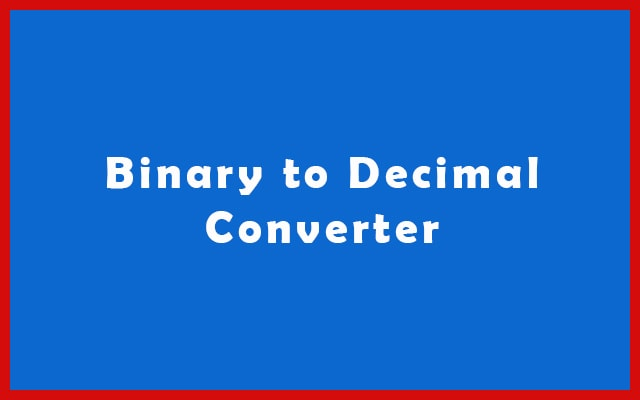# Binary to Decimal Converter and Calculator Online

Use Online Binary to Decimal Converter to convert binary language data into decimal. The conversion process is very simple. Just enter the values and hit the “Convert” button.

 Enter Binary Code: 2 Decimal Result: 10 Decimal From Signed 2’s Complement: 10 Hex Result: 16 Full Calculation:
Related

Binary is hard to understand. That’s why we use the Binary to Decimal Converter for the conversion process. The binary numbers are widely using in electronic circuits and in computer systems for data exchange.## Binary to Decimal Converter Online

Converting binary number into decimal will not be hard anymore with this binary to decimal calculator. Also, it is fast and accurate. The binary number is base 2. So, it Containing only two numbers 1 and 0. Decimal is base 10. So, we have to convert a base 2 into base 10 and as a result, we get decimal output.

#### Binary Numbers: The language of Computers

Computers don’t directly understand human instructions or normal language. So, Firstly, you have to convert them into binary. Because the computer only knows 0 and 1. Also, all the processes in a computer take place using binary. It is fundamental and most using numbering system in digital world.

1 is Logical True whereas 0 is Logical False. Also, 1 and 0 represent ON and OFF respectively.

Whenever there is 1 state. A maximum current flow occurs.
Whenever there is 0 state. Minimum current flow occurs.

Using this way, digital circuits are controlled.

“Gottfried Leibniz” was the person who introduced the binary numbering system. Along with binary, he reshaped the computing future. Even more, the article that you are reading is possible only because of it. Many electrical components are working to provide a continuous flow of data in the form of binary. Hence, binary numbers are so important.

#### Coding in Binary

In this, the instructions are provided in the binary form. But have you ever wondered? How instructions are transferred into systems? The answer is very simple. It is just because of “Programming”. Most importantly, machine-level programming is necessary.

The programming languages we learn and use today are very High-Level Programming Languages. For example, C++, JAVA, Python, Kotlin, etc. They are easy to understand by humans.

On the other hand, machine-level languages are completely different. They are not easy to understand and learn. So, we can use binary to do coding. This type of coding directly reaches the computer. So, there is no need for conversion.

Conversely, in High-Level Programming Languages, you first need to change it into machine language. Which is in the binary form. So, there are different applications designed for this purpose.

Compiler and Interpreter are using in this situation.

A Compiler converts the whole High-Level Programming Language script into Machine Language in one go.

An Interpreter converts one line of code at a time. It means, one line of High-Level Programming Language is converted into Machine Language.

In the case of compiling, you can see programming errors at the end of the compilation process. Even more, debugging is difficult in compiler.

In interpretation, if a line has an error then the process stops. Therefore, debugging is comparatively easier.

### Why Use Binary Numbering System?

If Binary is not easy to grasp. Then why we prefer it? Below are the major reasons behind it.

• Many logical circuits are using in a computer system. For example AND, OR, NOR, NAND, XOR, and many more. These are easy to control using two base signals(0 and 1). That’s why we go for binary.
• For reducing signal transmission from 10 to 2. We prefer binary signals. Also, it is necessary because the voltage fluctuation is common.
• To operate Optical and Magnetic components.

#### Advantages of Binary to Decimal Calculator

This tool is free to use as well as the user interface is very simple. The manual conversion takes too much time. Whereas, in this converter, it takes less than a second. Also, the accuracy is 100%. The method of binary conversion is complex. So, we have worked to make it easier for you.

### How to use Binary to Decimal Converter?

It’s an online tool. Therefore, get a device that could run the Internet. Then follow the instruction below.

1. First of all, open any web browser like Chrome, Safari, or Firefox.
2. Then, visit the Binary to Decimal Converter to convert binary codes into decimal.
3. After that, you can see an input option.
4. So, enter the binary numbers in an empty box. This binary number will convert into decimal.
5. Press the “Convert” button.
6. Lastly, for new conversion press “Reset” button.